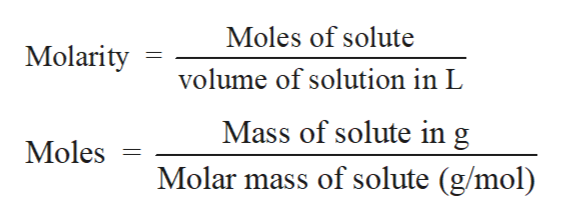# Given a solution of NaOH in Water that has a molarity of .04M, that contains .08 g of NaOH or .002 mol NaOH.  This is all in a 50 mL container.How many hydroxide ions (OH-) are present in this solution?

Question
34 views

Given a solution of NaOH in Water that has a molarity of .04M, that contains .08 g of NaOH or .002 mol NaOH.  This is all in a 50 mL container.

How many hydroxide ions (OH-) are present in this solution?

check_circle

star
star
star
star
star
1 Rating
Step 1

Solution stoichiometry involves the calculation of concentration of solutions in the given conditions of volumes, moles etc.

Step 2

Solution stoichiometry is mainly based on the calculation of moles and volumes. These two values are used to calculate the molarity of solution. The relation between moles, volume and molarity is as given below;help_outlineImage TranscriptioncloseMoles of solute Molarity volume of solution in L Mass of solute in g Moles Molar mass of solute (g/mol) fullscreen
Step 3

NaOH is a strong base which can easily ionized ...

### Want to see the full answer?

See Solution

#### Want to see this answer and more?

Solutions are written by subject experts who are available 24/7. Questions are typically answered within 1 hour.*

See Solution
*Response times may vary by subject and question.
Tagged in

### Solutions